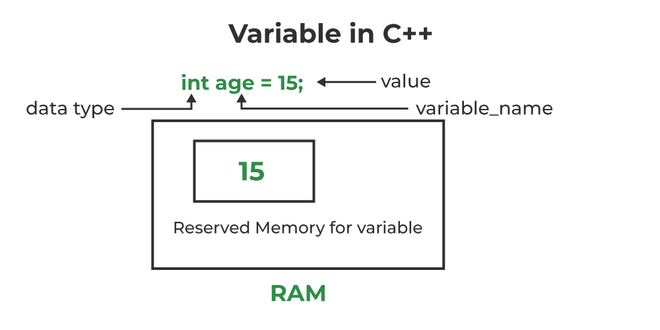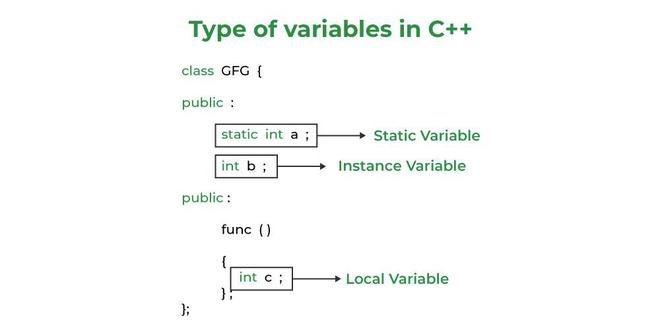Open in App
Not now

# C++ Variables

• Difficulty Level : Easy
• Last Updated : 16 Mar, 2023

Variables in C++ is a name given to a memory location. It is the basic unit of storage in a program.

• The value stored in a variable can be changed during program execution.
• A variable is only a name given to a memory location, all the operations done on the variable effects that memory location.
• In C++, all the variables must be declared before use.

## How to Declare Variables?

A typical variable declaration is of the form:

```// Declaring a single variable
type variable_name;

// Declaring multiple variables:
type variable1_name, variable2_name, variable3_name;```

A variable name can consist of alphabets (both upper and lower case), numbers, and the underscore ‘_’ character. However, the name must not start with a number.Initialization of a variable in C++

In the above diagram,

datatype: Type of data that can be stored in this variable.
variable_name: Name given to the variable.
value: It is the initial value stored in the variable.

Examples:

```// Declaring float variable
float simpleInterest;

// Declaring integer variable
int time, speed;

// Declaring character variable
char var;  ```

#### We can also provide values while declaring the variables as given below:

```int a=50,b=100;  //declaring 2 variable of integer type
float f=50.8;  //declaring 1 variable of float type
char c='Z';    //declaring 1 variable of char type   ```

## Rules For Declaring Variable

• The name of the variable contains letters, digits, and underscores.
• The name of the variable is case sensitive (ex Arr and arr both are different variables).
• The name of the variable does not contain any whitespace and special characters (ex #,\$,%,*, etc).
• All the variable names must begin with a letter of the alphabet or an underscore(_).
• We cannot used C++ keyword(ex float,double,class)as a variable name.

Valid variable names:

```int x;   //can be letters
int _yz; //can be underscores
int z40;//can be letters    ```

Invalid variable names:

```int 89; Should not be a number
int a b; //Should not contain any whitespace
int double;// C++ keyword CAN NOT BE USED  ```

## Difference Between Variable Declaration and Definition

The variable declaration refers to the part where a variable is first declared or introduced before its first use. A variable definition is a part where the variable is assigned a memory location and a value. Most of the time, variable declaration and definition are done together.
See the following C++ program for better clarification:

## C++

 `// C++ program to show difference between` `// definition and declaration of a ` `// variable` `#include ` `using` `namespace` `std;`   `int` `main()` `{` `    ``// this is declaration of variable a` `    ``int` `a;` `  `  `    ``// this is initialisation of a` `    ``a = 10;` `  `  `    ``// this is definition = declaration + initialisation` `    ``int` `b = 20;`   `    ``// declaration and definition` `    ``// of variable 'a123'` `    ``char` `a123 = ``'a'``;`   `    ``// This is also both declaration and definition` `    ``// as 'c' is allocated memory and` `    ``// assigned some garbage value.` `    ``float` `c;`   `    ``// multiple declarations and definitions` `    ``int` `_c, _d45, e;`   `    ``// Let us print a variable` `    ``cout << a123 << endl;`   `    ``return` `0;` `}`

Output

`a`

Time Complexity: O(1)

Space Complexity: O(1)

## Types of Variables

There are three types of variables based on the scope of variables in C++

• Local Variables
• Instance Variables
• Static VariablesTypes of Variables in C++

Let us now learn about each one of these variables in detail.

1. Local Variables: A variable defined within a block or method or constructor is called a local variable.
• These variables are created when entered into the block or the function is called and destroyed after exiting from the block or when the call returns from the function.
• The scope of these variables exists only within the block in which the variable is declared. i.e. we can access this variable only within that block.
• Initialization of Local Variable is Mandatory.
2. Instance Variables: Instance variables are non-static variables and are declared in a class outside any method, constructor, or block.
• As instance variables are declared in a class, these variables are created when an object of the class is created and destroyed when the object is destroyed.
• Unlike local variables, we may use access specifiers for instance variables. If we do not specify any access specifier then the default access specifier will be used.
• Initialization of Instance Variable is not Mandatory.
• Instance Variable can be accessed only by creating objects.
3. Static Variables: Static variables are also known as Class variables.
• These variables are declared similarly as instance variables, the difference is that static variables are declared using the static keyword within a class outside any method constructor or block.
• Unlike instance variables, we can only have one copy of a static variable per class irrespective of how many objects we create.
• Static variables are created at the start of program execution and destroyed automatically when execution ends.
• Initialization of Static Variable is not Mandatory. Its default value is 0
• If we access the static variable like the Instance variable (through an object), the compiler will show the warning message and it won’t halt the program. The compiler will replace the object name with the class name automatically.
• If we access the static variable without the class name, the Compiler will automatically append the class name.

## Instance Variable Vs Static Variable

• Each object will have its own copy of the instance variable whereas We can only have one copy of a static variable per class irrespective of how many objects we create.
• Changes made in an instance variable using one object will not be reflected in other objects as each object has its own copy of the instance variable. In the case of static, changes will be reflected in other objects as static variables are common to all objects of a class.
• We can access instance variables through object references and Static Variables can be accessed directly using the class name.
• The syntax for static and instance variables:
```class Example
{
static int a; // static variable
int b;        // instance variable
}```

My Personal Notes arrow_drop_up
Related Articles